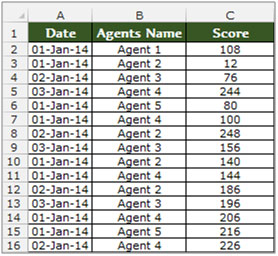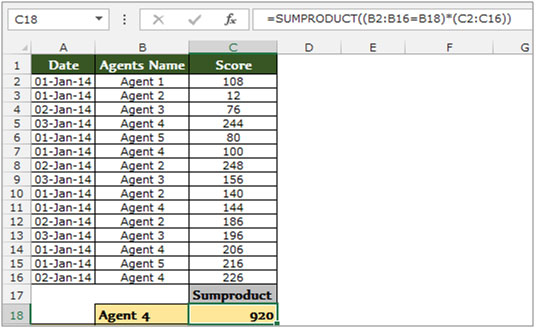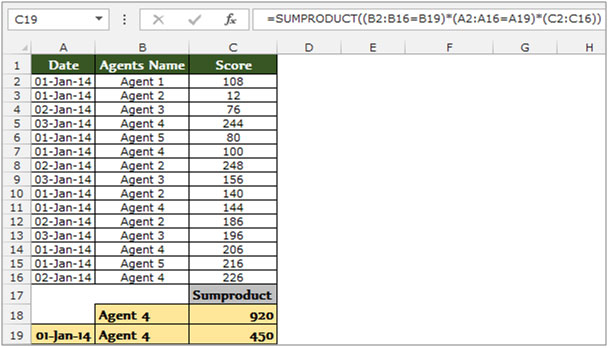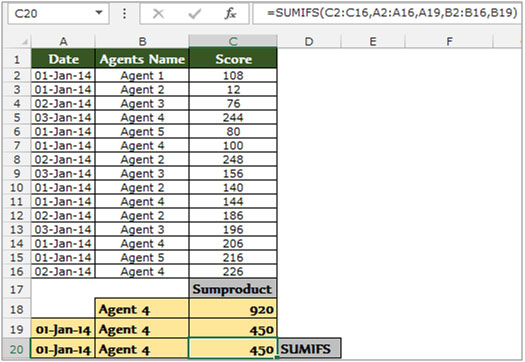How to Use Sumproduct with Multiple Criteria in Excel 2010

Sum product in Excel is a multipurpose function which will provide different results depending on the way the function’s arguments are entered. The purpose of “Sum product” is to multiply two or more ranges and then sum them in Microsoft Excel 2010.

Syntax of “SUMPRODUCT” function:

=sum product (array1, array2, array3……….).

Let’s understand with a simple exercise how we can use theSumproduct function with multiple criteria.

We have table in Range “A2 to C16” which contains the Agents score data. Column A contains date, Column B Agents Name and Column C contains score. Now we need to calculate the total score of Agent 4 in cell C18. Let’s see how we can use the sumproduct function to return this value..• Select the cell C18, and write the formula in the cell.
• =SUMPRODUCT((B2:B16=B18)*(C2:C16))
• Press Enter on the keyboard.
• The function will return the score value of Agent 4You can also use the SUMPRODUCT function for multiple criteria.

Let’s say, now we require the total score of Agent 4 only for 1stJan’2014.

• Select the cell C19, and write the formula in the cell.
• =SUMPRODUCT((B2:B16=B19)*(A2:A16=A19)*(C2:C16))
• Press Enter on the keyboard.
• The function will return the score value of Agent 4 as on 1st-Jan-2014.Note: - You can use the SUMIFs function instead of SUMPRODUCT function for a better result.

• Select the cell C20, and write the formula in the cell.
• =SUMIFS(C2:C16,A2:A16,A19,B2:B16,B19)
• Press Enter on the keyboard.
• The function will return the score value of Agent 4 as on 1st-Jan-2014.This is how we can arrive at the same answer using both the SUMIFS and the SUMPRODUCT functions.
.

Terms and Conditions of use

The applications/code on this site are distributed as is and without warranties or liability. In no event shall the owner of the copyrights, or the authors of the applications/code be liable for any loss of profit, any problems or any damage resulting from the use or evaluation of the applications/code.